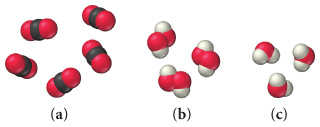# Problem: Write a ratio showing the relationship between the amounts of each element for each of the following:(b) A) 2H:OB) 2H:2O C) 3H:OD) H:2O

###### FREE Expert Solution

O → red sphere

H → white sphere###### Problem Details

Write a ratio showing the relationship between the amounts of each element for each of the following:(b)

A) 2H:O

B) 2H:2O

C) 3H:O

D) H:2O